### Optical Illusion #2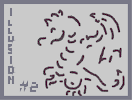Hover over the thumbnail for a full-size version.

Author Dragon_Moon author:dragon_moon rated test 2006-12-02 4 by 30 people. \$Optical Illusion 2#Dragon_Moon#none#000000000000000000000000OO0QPQPQPEDPOONQPJQ00000000000Q9000006Q9QQ000000000000000000000000000000000000000000000GPI00000000000000000000GEI0000000000000000000007O00000000000000000000NNN000000000000000000000Q0000000000000000000000000000000000000000000000000000000000000000000000000000000000000000000000000000000000000000000000000000000000000000000000000000000000000000000000000000000000000000000000000000000000000000000000000000000000000000000000000000000000000000000000000000000000000000000000000000000000000000000000000000000000000000000000000000000000000000000000000000000000000000000000000000000000000000000000000000000000000000000000000000000000000000000000000000000000000000000000000000000000000000000000000000|10^243,270!10^239,268!10^235,266!10^231,261!10^231,258!10^231,253!10^231,248!10^233,242!10^235,239!10^237,236!10^238,234!10^242,231!10^248,230!10^253,230!10^257,230!10^262,230!10^267,230!10^270,229!10^272,228!10^309,263!10^309,259!10^311,255!10^314,252!10^316,248!10^319,246!10^322,245!10^328,243!10^331,243!10^338,243!10^348,243!10^353,242!10^359,240!10^364,238!10^367,235!10^372,232!10^339,244!10^344,243!10^348,242!10^352,240!10^357,234!10^363,230!10^371,229!10^373,227!10^376,224!10^379,220!10^381,213!10^382,204!10^382,200!10^382,195!10^382,193!10^381,190!10^377,186!10^377,186!10^379,187!10^381,194!10^381,198!10^380,204!10^374,213!10^372,218!10^369,222!10^369,228!10^373,224!10^374,215!10^378,209!10^382,201!10^383,195!10^382,205!10^375,216!10^360,234!10^354,237!10^347,238!10^339,238!10^334,238!10^331,241!10^322,242!10^332,240!10^342,239!10^319,171!10^316,173!10^313,175!10^311,180!10^309,184!10^309,190!10^309,191!10^311,185!10^312,181!10^313,173!10^315,171!10^323,171!10^325,173!10^329,176!10^325,174!10^319,174!10^311,181!10^311,186!10^311,188!10^363,238!10^368,234!10^371,226!10^376,216!10^379,211!10^380,210!10^286,154!10^286,149!10^284,144!10^282,139!10^280,135!10^277,129!10^273,123!10^270,120!10^269,119!10^273,118!10^276,120!10^278,126!10^285,140!10^274,117!10^283,117!10^287,118!10^291,118!10^298,119!10^305,124!10^307,124!10^300,120!10^293,117!10^289,117!10^281,116!10^279,116!10^311,90!10^311,94!10^311,96!10^311,93!10^311,89!10^312,87!10^315,86!10^322,86!10^327,86!10^332,86!10^336,87!10^339,89!10^340,90!10^342,92!10^336,89!10^326,87!10^322,87!10^318,87!10^349,97!10^349,97!10^348,95!10^345,94!10^352,74!10^356,73!10^358,70!10^360,66!10^361,65!10^361,65!10^364,67!10^367,70!10^370,73!10^372,76!10^372,82!10^372,87!10^372,89!10^373,85!10^373,78!10^356,69!10^356,69!10^412,103!10^415,103!10^420,104!10^423,104!10^429,104!10^435,104!10^439,107!10^442,108!10^446,111!10^453,117!10^456,120!10^464,126!10^466,127!10^461,125!10^454,120!10^453,118!10^448,113!10^442,108!10^437,105!10^432,102!10^428,99!10^476,141!10^473,140!10^470,135!10^467,131!10^464,127!10^469,129!10^472,136!10^473,139!10^476,144!10^477,150!10^478,152!10^478,145!10^427,100!10^424,100!10^420,100!10^414,101!10^411,101!10^459,125!10^451,114!10^444,107!10^415,214!10^417,217!10^419,219!10^425,220!10^427,220!10^434,217!10^437,215!10^443,211!10^447,206!10^449,198!10^449,198!10^444,208!10^441,212!10^436,214!10^423,217!10^450,202!10^450,202!10^413,244!10^413,249!10^414,253!10^417,254!10^420,254!10^430,254!10^437,253!10^445,250!10^449,247!10^451,245!10^447,248!10^442,250!10^432,252!10^423,253!10^399,285!10^399,287!10^399,294!10^399,299!10^401,300!10^407,303!10^412,307!10^418,310!10^426,310!10^437,310!10^441,310!10^433,312!10^425,312!10^421,312!10^413,308!10^407,304!10^403,301!10^371,320!10^366,324!10^366,331!10^367,339!10^370,345!10^377,350!10^392,357!10^396,358!10^387,357!10^381,356!10^378,354!10^374,351!10^371,347!10^370,341!10^370,330!10^370,320!10^472,375!10^473,375!10^480,371!10^484,365!10^488,357!10^492,350!10^496,343!10^498,335!10^498,335!10^494,342!10^491,353!10^486,360!10^477,370!10^473,375!10^485,369!10^529,234!10^529,231!10^533,228!10^537,223!10^541,220!10^550,220!10^558,220!10^564,219!10^571,215!10^579,210!10^581,207!10^584,200!10^584,193!10^584,187!10^526,235!10^524,233!10^521,225!10^521,221!10^521,216!10^522,221!10^527,231!10^529,233!10^532,224!10^539,223!10^551,223!10^562,222!10^574,220!10^578,212!10^583,203!10^583,197!10^541,221!10^552,221!10^560,221!10^567,220!10^571,218!10^575,216!10^566,104!10^561,102!10^558,99!10^556,97!10^551,95!10^545,93!10^543,93!10^545,91!10^548,89!10^552,86!10^557,84!10^564,84!10^568,84!10^567,84!10^561,84!10^576,118!10^575,122!10^575,125!10^577,126!10^583,129!10^590,129!10^592,127!10^593,125!10^617,82!10^620,84!10^629,85!10^636,86!10^644,90!10^650,93!10^657,95!10^661,97!10^671,104!10^677,110!10^683,116!10^688,123!10^691,127!10^695,135!10^701,143!10^705,152!10^707,156!10^708,164!10^710,171!10^712,179!10^714,183!10^715,189!10^715,188!10^712,177!10^710,169!10^708,158!10^707,148!10^700,139!10^693,131!10^690,124!10^684,118!10^677,109!10^672,103!10^666,99!10^662,96!10^658,93!10^653,91!10^646,89!10^639,87!10^631,86!10^624,84!10^621,83!10^621,83!10^494,356!10^495,348!10^497,342!10^498,336!10^496,353!10^488,365!10^498,350!10^498,350!10^500,344!10^500,341!10^500,336!10^485,367!10^481,371!10^480,374!10^476,378!10^475,378!10^472,380!10^470,382!10^466,384!10^465,384!10^464,387!10^464,387!10^468,385!10^472,381!10^477,376!10^481,371!10^492,360!10^492,362!10^492,362!10^494,356!10^494,356!10^721,340!10^721,336!10^721,331!10^722,326!10^723,322!10^723,317!10^723,312!10^724,307!10^724,301!10^724,295!10^724,295!10^723,310!10^723,316!10^723,323!10^723,327!10^723,336!10^717,312!10^716,309!10^711,304!10^706,303!10^701,303!10^693,303!10^685,303!10^682,306!10^682,307!10^687,304!10^694,303!10^703,303!10^714,305!10^663,360!10^663,357!10^665,353!10^665,350!10^666,346!10^661,351!10^661,358!10^659,363!10^659,369!10^664,360!10^667,350!10^668,343!10^668,338!10^674,232!10^675,230!10^675,222!10^675,215!10^675,208!10^675,202!10^671,193!10^670,187!10^669,181!10^666,175!10^664,173!10^670,184!10^675,196!10^677,204!10^678,213!10^678,222!10^613,357!10^616,357!10^620,354!10^629,353!10^633,353!10^624,353!10^616,356!10^611,360!10^611,361!10^617,355!10^595,378!10^597,376!10^598,373!10^600,367!10^601,363!10^602,359!10^603,354!10^606,349!10^607,343!10^610,337!10^612,332!10^613,327!10^616,322!10^606,349!10^606,345!10^607,340!10^607,334!10^610,327!10^613,320!10^613,316!10^615,311!10^617,310!10^615,312!10^615,322!10^614,326!10^618,305!10^618,303!10^613,213!10^612,216!10^598,239!10^588,246!10^582,248!10^581,249!10^589,243!10^594,237!10^605,234!10^611,219!10^613,213!10^608,223!10^606,231!10^600,236!10^597,241!10^595,243!10^607,226!10^608,226!10^603,229!10^601,235!10^597,238!10^600,239!10^607,231!10^609,223!10^607,146!10^611,148!10^612,150!10^620,157!10^620,158!10^614,150!10^610,145!10^624,163!10^624,166!10^624,165!10^621,158!10^617,155!10^619,300!10^621,296!10^624,292!10^625,285!10^637,200!10^633,188!10^629,180!10^626,169!10^626,169!10^629,173!10^631,181!10^631,187!10^634,194!10^635,197!10^636,203!10^636,198!10^633,187!10^630,181!10^626,173!10^613,114!10^615,113!10^618,114!10^621,116!10^628,119!10^632,120!10^636,124!10^632,119!10^543,275!10^534,277!10^528,281!10^524,285!10^525,284!10^530,277!10^535,274!10^548,274!10^491,244!10^485,246!10^484,248!10^485,256!10^487,257!10^486,253!10^486,247!10^489,239!10^495,236!10^501,235!10^501,235!10^424,433!10^425,433!10^430,432!10^434,432!10^438,432!10^449,433!10^459,436!10^461,441!10^455,438!10^450,435!10^439,435!10^433,435!10^439,434!10^446,434!10^390,499!10^391,505!10^392,511!10^395,517!10^402,523!10^408,526!10^414,530!10^421,532!10^427,532!10^436,532!10^443,532!10^449,532!10^452,531!10^457,529!10^461,526!10^462,525!10^455,527!10^446,530!10^437,531!10^428,532!10^425,532!10^419,529!10^410,525!10^402,520!10^396,513!10^391,505!10^428,497!10^434,499!10^440,503!10^432,501!10^467,522!10^470,521!10^472,518!10^474,515!10^477,512!10^478,507!10^481,501!10^483,496!10^484,488!10^487,480!10^487,477!10^486,484!10^510,539!10^510,541!10^510,547!10^510,552!10^509,555!10^509,555!10^507,558!10^475,545!10^473,549!10^472,556!10^473,559!10^478,566!10^480,568!10^482,569!10^484,571!10^487,573!10^487,574!10^478,565!10^478,557!10^478,551!10^451,552!10^447,552!10^441,554!10^439,560!10^439,567!10^439,574!10^439,570!10^439,562!10^417,548!10^415,548!10^406,552!10^403,558!10^403,566!10^403,565!10^405,553!10^407,549!10^391,497!10^393,492!10^394,486!10^394,479!10^394,476!10^390,484!10^390,494!10^390,502!10^391,496!10^391,486!10^391,479!10^345,368!10^345,371!10^345,381!10^346,387!10^350,394!10^354,400!10^355,403!10^360,404!10^337,435!10^340,440!10^347,447!10^354,451!10^361,451!10^369,451!10^377,451!10^381,449!10^379,451!10^372,453!10^361,454!10^355,454!10^350,450!10^343,443!10^362,488!10^366,489!10^371,489!10^372,488!10^363,488!10^374,404!10^370,404!10^367,404!10^364,404!10^360,402!10^354,397!10^349,390!10^346,384!10^344,376!10^318,405!10^314,405!10^311,406!10^306,406!10^298,406!10^290,408!10^286,410!10^277,414!10^270,418!10^267,422!10^265,429!10^265,437!10^267,443!10^271,445!10^267,444!10^264,439!10^264,428!10^268,419!10^277,411!10^290,405!10^296,405!10^277,496!10^277,493!10^277,488!10^278,484!10^279,481!10^282,478!10^287,477!10^294,478!10^302,478!10^307,476!10^312,471!10^319,462!10^319,462!10^314,467!10^277,496!10^277,502!10^277,505!10^278,506!10^278,503!10^315,499!10^324,496!10^328,496!10^333,491!10^336,488!10^335,488!10^326,493!10^317,493!10^319,499!10^311,499!10^305,499!10^300,504!10^300,507!10^300,503!10^303,496!10^312,496!10^279,513!10^279,519!10^281,523!10^283,526!10^283,526!10^320,466!10^321,461!10^322,457!10^323,453!10^323,453!10^317,454!10^310,455!10^306,455!10^304,455!10^530,431!10^544,438!10^544,439!10^550,440!10^544,437!10^533,430!10^531,429!10^529,429!10^528,428!10^521,425!10^518,424!10^515,421!10^508,414!10^508,414!10^548,441!10^542,437!10^537,434!10^535,432!10^528,427!10^520,420!10^511,414!10^507,411!10^502,409!10^502,409!10^553,444!10^560,445!10^565,449!10^573,452!10^579,455!10^584,460!10^589,464!10^594,472!10^598,476!10^602,483!10^603,486!10^607,491!10^611,494!10^614,500!10^616,502!10^631,511!10^628,510!10^626,509!10^622,506!10^620,505!10^610,537!10^606,536!10^602,533!10^595,527!10^588,521!10^586,519!10^585,519!10^595,526!10^605,534!10^605,534!10^595,525!10^584,517!10^540,480!10^535,478!10^529,475!10^524,475!10^520,473!10^515,473!10^513,473!10^513,473!10^526,474!10^532,476!10^697,511!10^703,507!10^705,503!10^705,494!10^705,485!10^701,484!10^696,484!10^690,487!10^693,484!10^704,482!10^710,483!10^712,494!10^710,502!10^704,509!10^697,510!10^690,514!10^685,517!10^677,518!10^679,518!10^689,516!10^699,506!10^721,534!10^723,532!10^726,528!10^731,523!10^734,520!10^735,517!10^737,514!10^740,509!10^742,506!10^742,501!10^742,494!10^742,489!10^742,481!10^742,473!10^742,469!10^741,464!10^740,459!10^740,462!10^742,476!10^742,486!10^742,494!10^742,505!10^736,514!10^711,424!10^717,426!10^720,429!10^709,422!10^702,422!10^706,422!10^727,433!10^725,432!10^719,429!10^705,427!10^707,430!10^708,433!10^709,437!10^709,439!10^709,441!10^709,444!10^709,450!10^709,450!10^644,545!10^642,545!10^636,545!10^632,545!10^627,545!10^622,545!10^615,545!10^608,542!10^601,536!10^593,528!10^243,268!10^242,268!10^241,268!10^241,268!10^240,268!10^239,268!10^237,265!10^236,263!10^235,260!10^233,258!10^232,257!10^231,260!10^233,260!10^233,261!10^234,261!10^232,256!10^232,254!10^231,253!10^231,251!10^231,251!10^231,250!10^231,248!10^231,246!10^231,243!10^233,241!10^234,239!10^236,238!10^236,237!10^237,236!10^238,236!10^240,235!10^240,235!10^241,234!10^242,234!10^242,233!10^244,232!10^245,231!10^246,230!10^248,230!10^250,230!10^253,230!10^253,230!10^256,230!10^257,230!10^259,230!10^262,230!10^266,230!10^267,230!10^269,230!10^270,229!10^270,229!10^265,229!10^261,229!10^259,231!10^258,231!10^256,231!10^243,268!10^242,268!10^309,192!10^309,191!10^309,191!10^309,189!10^309,188!10^309,184!10^309,180!10^309,178!10^311,176!10^313,175!10^315,173!10^316,172!10^320,172!10^321,172!10^323,173!10^325,174!10^326,174!10^327,174!10^328,174!10^328,174!10^308,260!10^309,260!10^310,259!10^310,256!10^310,254!10^311,252!10^312,251!10^313,250!10^315,248!10^317,246!10^320,245!10^321,245!10^322,244!10^324,244!10^326,244!10^327,244!10^327,244!10^328,243!10^328,243!10^330,242!10^332,242!10^335,241!10^339,241!10^342,241!10^344,241!10^345,241!10^349,241!10^353,241!10^355,240!10^357,239!10^358,238!10^360,237!10^361,236!10^362,234!10^363,234!10^364,232!10^366,231!10^368,228!10^370,226!10^371,224!10^372,222!10^373,220!10^373,220!10^376,218!10^377,215!10^377,212!10^378,208!10^381,206!10^381,203!10^382,200!10^382,196!10^382,193!10^382,191!10^382,191!10^381,188!10^285,152!10^285,150!10^285,150!10^285,148!10^285,146!10^285,145!10^284,142!10^283,141!10^282,138!10^280,135!10^277,131!10^275,129!10^275,129!10^274,125!10^272,123!10^272,123!10^277,130!10^277,130!10^306,123!10^303,122!10^303,122!10^301,121!10^301,121!10^298,119!10^294,118!10^291,118!10^288,118!10^284,118!10^282,118!10^280,118!10^311,96!10^311,96!10^311,93!10^311,90!10^311,87!10^314,85!10^316,84!10^319,84!10^323,84!10^326,84!10^328,85!10^331,85!10^334,85!10^336,86!10^337,88!10^340,89!10^342,92!10^342,93!10^344,95!10^346,95!10^372,87!10^372,83!10^372,80!10^372,76!10^371,72!10^367,67!10^362,64!10^360,63!10^360,63!10^360,65!10^359,67!10^358,69!10^356,71!10^355,74!10^355,75!10^478,149!10^476,147!10^475,143!10^474,140!10^472,137!10^470,134!10^467,129!10^463,124!10^462,122!10^457,119!10^457,118!10^449,112!10^447,110!10^447,110!10^444,107!10^442,105!10^440,104!10^438,104!10^436,103!10^434,103!10^432,103!10^425,103!10^422,103!10^422,103!10^450,199!10^449,200!10^449,204!10^449,205!10^446,206!10^445,209!10^443,211!10^442,212!10^439,213!10^437,216!10^435,217!10^432,219!10^430,221!10^430,221!10^427,221!10^424,221!10^423,221!10^420,218!10^417,217!10^415,215!10^414,215!10^414,215!10^416,218!10^415,218!10^415,217!10^415,217!10^415,217!10^564,103!10^563,103!10^561,101!10^558,99!10^553,96!10^548,94!10^547,93!10^546,93!10^546,89!10^548,88!10^549,87!10^552,85!10^555,83!10^558,83!10^563,83!10^565,83!10^567,83!10^554,98!10^552,96!10^593,126!10^591,127!10^591,129!10^589,131!10^586,131!10^585,131!10^582,128!10^579,126!10^578,123!10^577,121!10^577,121!10^577,121!10^634,122!10^633,121!10^629,119!10^627,118!10^625,117!10^623,117!10^620,116!10^618,116!10^616,116!10^633,120!10^633,121!10^714,189!10^714,186!10^714,183!10^714,181!10^713,180!10^712,178!10^712,176!10^712,174!10^712,171!10^711,169!10^710,166!10^709,163!10^709,163!10^709,162!10^707,159!10^706,156!10^706,154!10^705,153!10^705,151!10^705,149!10^705,149!10^704,146!10^703,144!10^702,140!10^700,137!10^698,135!10^696,134!10^695,132!10^695,132!10^693,129!10^693,129!10^691,127!10^687,123!10^686,121!10^684,118!10^683,116!10^680,112!10^680,112!10^676,107!10^675,106!10^674,104!10^673,104!10^671,102!10^670,101!10^668,100!10^666,99!10^662,96!10^660,95!10^659,95!10^657,94!10^655,93!10^651,92!10^649,91!10^646,90!10^642,89!10^639,88!10^634,86!10^634,86!10^630,86!10^627,85!10^622,84!10^621,84!10^620,84!10^618,83!10^618,83!10^684,114!10^681,112!10^674,229!10^675,228!10^676,227!10^676,224!10^677,221!10^677,219!10^677,217!10^677,215!10^677,214!10^677,214!10^677,213!10^677,209!10^677,207!10^676,203!10^676,200!10^675,197!10^675,195!10^675,193!10^673,190!10^672,189!10^672,188!10^671,184!10^671,181!10^668,178!10^667,175!10^666,173!10^666,173!10^720,329!10^721,338!10^721,337!10^721,333!10^721,328!10^721,324!10^721,318!10^723,313!10^723,312!10^723,308!10^723,303!10^723,300!10^723,298!10^723,296!10^724,294!10^724,293!10^722,316!10^719,311!10^716,308!10^713,307!10^710,305!10^706,303!10^703,302!10^696,303!10^693,303!10^691,305!10^688,306!10^684,306!10^684,306!10^691,304!10^694,302!10^701,302!10^702,302!10^658,370!10^658,366!10^659,363!10^661,361!10^662,357!10^663,352!10^663,347!10^664,345!10^667,342!10^668,341!10^669,341!10^669,341!10^660,367!10^660,369!10^669,340!10^632,352!10^631,352!10^629,352!10^623,352!10^616,352!10^613,355!10^610,358!10^607,361!10^602,364!10^601,369!10^599,372!10^599,372!10^601,366!10^601,357!10^603,347!10^604,343!10^605,339!10^606,337!10^609,333!10^611,331!10^613,327!10^615,323!10^616,321!10^616,318!10^617,313!10^617,308!10^618,304!10^618,300!10^620,296!10^622,292!10^624,288!10^626,286!10^624,290!10^601,352!10^602,352!10^606,349!10^606,345!10^606,341!10^606,334!10^609,329!10^611,324!10^611,324!10^636,202!10^636,201!10^635,197!10^635,193!10^633,189!10^631,185!10^630,181!10^629,178!10^628,175!10^628,172!10^626,168!10^625,166!10^623,162!10^622,161!10^621,159!10^619,156!10^618,154!10^617,152!10^613,149!10^611,146!10^610,146!10^608,143!10^581,249!10^581,249!10^582,248!10^583,247!10^584,246!10^588,245!10^591,244!10^592,244!10^593,242!10^594,241!10^596,240!10^599,239!10^601,238!10^603,235!10^605,233!10^606,230!10^606,228!10^608,225!10^609,223!10^610,222!10^611,220!10^612,218!10^612,216!10^613,214!10^613,214!10^527,234!10^526,233!10^526,233!10^525,231!10^524,228!10^523,226!10^522,223!10^521,221!10^520,218!10^520,218!10^520,216!10^520,216!10^521,221!10^522,225!10^523,227!10^524,227!10^528,227!10^530,228!10^535,225!10^537,222!10^540,221!10^540,221!10^542,220!10^546,220!10^548,220!10^550,220!10^554,220!10^557,220!10^559,220!10^564,220!10^568,220!10^570,218!10^571,216!10^573,215!10^575,214!10^576,213!10^578,211!10^580,210!10^581,209!10^582,206!10^583,204!10^584,202!10^584,200!10^585,197!10^585,196!10^585,193!10^585,193!10^585,191!10^585,191!10^585,189!10^585,188!10^585,188!10^485,256!10^485,254!10^485,252!10^485,249!10^485,246!10^487,244!10^488,243!10^489,242!10^489,241!10^490,240!10^492,238!10^493,237!10^494,236!10^497,236!10^500,236!10^500,236!10^500,236!10^450,243!10^450,245!10^449,247!10^446,249!10^443,251!10^440,252!10^438,252!10^435,252!10^433,252!10^431,253!10^429,254!10^427,254!10^424,254!10^424,254!10^422,254!10^420,254!10^418,254!10^416,252!10^414,251!10^414,250!10^411,247!10^411,245!10^411,245!10^411,245!10^411,245!10^411,245!10^411,247!10^411,247!10^524,285!10^525,285!10^525,284!10^527,282!10^528,281!10^529,280!10^530,280!10^530,278!10^531,277!10^533,276!10^533,276!10^535,275!10^537,275!10^540,275!10^540,275!10^542,275!10^545,275!10^545,275!10^526,282!10^526,282!10^440,310!10^438,310!10^435,312!10^434,312!10^431,312!10^429,312!10^427,312!10^426,312!10^424,311!10^423,311!10^420,311!10^419,311!10^417,310!10^416,309!10^413,308!10^411,306!10^409,305!10^406,303!10^406,303!10^404,300!10^404,300!10^401,297!10^401,296!10^400,293!10^400,292!10^399,292!10^399,289!10^398,288!10^398,288!10^398,288!10^396,358!10^395,358!10^393,358!10^391,358!10^389,358!10^387,357!10^386,357!10^384,356!10^382,356!10^380,354!10^378,351!10^375,348!10^375,348!10^373,347!10^371,345!10^371,345!10^371,345!10^369,341!10^368,339!10^368,339!10^368,337!10^367,335!10^367,335!10^367,332!10^367,332!10^367,329!10^367,326!10^369,324!10^370,323!10^371,322!10^371,322!10^374,406!10^374,406!10^371,405!10^369,404!10^367,404!10^364,404!10^362,403!10^359,402!10^359,401!10^354,396!10^353,395!10^353,395!10^351,393!10^351,392!10^350,389!10^349,389!10^349,387!10^349,385!10^349,385!10^348,383!10^347,381!10^346,380!10^346,379!10^345,377!10^345,376!10^345,375!10^345,372!10^345,372!10^345,368!10^345,368!10^270,445!10^269,443!10^268,441!10^267,439!10^267,438!10^266,435!10^266,435!10^266,432!10^266,430!10^266,429!10^267,426!10^267,423!10^268,419!10^270,417!10^271,415!10^273,414!10^275,412!10^278,410!10^283,409!10^284,409!10^288,408!10^289,408!10^291,406!10^293,405!10^296,405!10^300,405!10^304,405!10^307,405!10^309,405!10^313,405!10^315,405!10^316,405!10^316,405!10^282,526!10^282,525!10^281,522!10^280,520!10^279,517!10^279,515!10^278,514!10^278,513!10^278,511!10^278,508!10^278,506!10^278,503!10^278,502!10^278,499!10^278,495!10^278,494!10^278,491!10^278,489!10^278,486!10^279,484!10^279,480!10^280,478!10^281,477!10^283,476!10^287,476!10^291,476!10^292,476!10^293,476!10^297,476!10^297,476!10^300,476!10^302,476!10^305,476!10^308,475!10^309,474!10^311,472!10^313,470!10^316,467!10^321,462!10^322,460!10^322,457!10^322,454!10^319,453!10^315,454!10^312,455!10^307,455!10^306,455!10^306,455!10^305,455!10^298,506!10^299,504!10^300,502!10^301,500!10^302,499!10^305,498!10^309,498!10^314,499!10^317,499!10^320,497!10^322,496!10^326,496!10^329,495!10^331,493!10^333,492!10^334,491!10^381,447!10^382,450!10^378,451!10^377,451!10^373,451!10^371,451!10^370,451!10^369,451!10^364,451!10^359,451!10^358,451!10^357,451!10^356,451!10^353,451!10^352,451!10^351,450!10^350,448!10^346,444!10^345,443!10^343,441!10^343,441!10^340,437!10^338,436!10^338,436!10^340,442!10^343,443!10^344,444!10^348,444!10^460,440!10^458,440!10^454,438!10^454,438!10^453,437!10^450,436!10^448,436!10^446,435!10^442,435!10^440,435!10^436,435!10^436,435!10^433,434!10^430,530!10^424,530!10^419,530!10^415,528!10^411,525!10^408,524!10^407,524!10^405,522!10^402,519!10^400,518!10^398,515!10^397,515!10^395,512!10^393,508!10^392,505!10^391,502!10^391,499!10^391,497!10^390,495!10^390,492!10^390,490!10^390,485!10^390,480!10^393,477!10^370,488!10^368,488!10^366,488!10^366,488!10^366,488!10^440,503!10^436,502!10^434,500!10^431,498!10^434,501!10^435,504!10^438,504!10^424,529!10^429,529!10^430,529!10^431,529!10^433,529!10^437,531!10^437,531!10^440,531!10^443,531!10^446,531!10^450,531!10^454,531!10^455,529!10^458,527!10^460,526!10^462,525!10^464,524!10^467,522!10^468,521!10^469,519!10^472,518!10^475,514!10^475,514!10^476,513!10^478,510!10^479,507!10^481,504!10^481,502!10^482,500!10^483,495!10^483,495!10^484,491!10^486,488!10^486,488!10^487,482!10^487,479!10^487,479!10^487,479!10^401,566!10^401,564!10^401,560!10^401,556!10^403,553!10^405,551!10^406,550!10^408,549!10^411,548!10^412,548!10^415,548!10^439,571!10^439,568!10^439,567!10^439,565!10^439,561!10^439,559!10^439,558!10^442,556!10^443,555!10^445,553!10^447,552!10^448,552!10^451,552!10^485,572!10^483,571!10^482,569!10^480,567!10^476,562!10^474,559!10^473,557!10^473,555!10^473,552!10^474,548!10^475,546!10^475,546!10^507,557!10^508,557!10^509,555!10^509,553!10^509,550!10^509,546!10^510,543!10^511,542!10^511,541!10^511,540!10^512,532!10^513,531!10^513,528!10^512,526!10^512,525!10^512,522!10^511,520!10^511,519!10^511,518!10^511,517!10^510,516!10^542,480!10^540,480!10^538,479!10^535,478!10^532,478!10^529,477!10^524,475!10^521,475!10^517,475!10^513,475!10^424,433!10^426,432!10^427,432!10^430,432!10^432,432!10^630,509!10^628,509!10^627,509!10^623,506!10^623,505!10^621,504!10^618,501!10^614,497!10^614,497!10^611,493!10^611,493!10^608,488!10^608,488!10^605,486!10^605,486!10^601,480!10^601,480!10^599,477!10^598,477!10^598,476!10^593,471!10^593,471!10^593,470!10^590,466!10^590,466!10^590,465!10^587,462!10^586,461!10^582,458!10^579,454!10^577,453!10^574,451!10^571,450!10^570,449!10^567,449!10^564,449!10^563,448!10^556,444!10^554,443!10^549,441!10^549,441!10^522,422!10^521,421!10^516,418!10^512,416!10^511,415!10^508,413!10^506,410!10^505,410!10^505,410!10^644,545!10^642,545!10^639,545!10^636,545!10^633,545!10^629,545!10^626,545!10^626,545!10^623,545!10^616,544!10^612,543!10^611,543!10^606,539!10^604,538!10^599,532!10^597,530!10^592,524!10^678,518!10^681,518!10^684,518!10^686,517!10^688,516!10^691,514!10^694,512!10^696,511!10^699,508!10^702,506!10^703,505!10^705,502!10^708,499!10^709,496!10^709,493!10^709,491!10^709,488!10^709,484!10^706,482!10^701,480!10^698,480!10^693,482!10^693,483!10^707,448!10^708,443!10^708,437!10^708,433!10^707,428!10^710,427!10^715,427!10^717,428!10^718,430!10^720,430!10^721,431!10^723,532!10^724,532!10^725,531!10^727,529!10^729,526!10^729,525!10^731,523!10^732,521!10^732,520!10^733,518!10^733,518!10^735,516!10^736,514!10^737,512!10^738,509!10^740,505!10^741,502!10^741,498!10^741,495!10^741,492!10^741,486!10^741,482!10^741,479!10^741,475!10^741,471!10^741,467!10^741,463!10^741,463# What is it? itsa wyvern (dragon with wings as arms) If u see it, look at this picture again. There are only black lines, no wyvern. all lines are disconected. but ur brain connects them and makes a complete picture of it. do u know what i mean? just try it. hope ya have fun =3

## Other maps by this author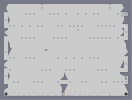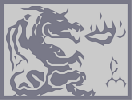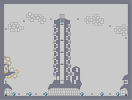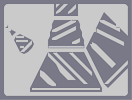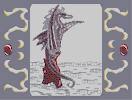Escape from rising lava Honorably Dragon He's pointing at you Traffic City Crome Cubes Afternoondragon 02 Step 2

Pages: (0)

### I see a wyvern

with a cute bellybutton. :D

:P

### lol remote

i can see why you say that, but when I look at this in full screen, I see either a dragon or a face... 4

### of course...

principal ervery pic is an illusion. But some are so perfec, u wont notice it. but, its still funny to show the simplest example. But if u dont know the thing, nemetacyst sad, uve to learn it. and these pictures are the best way to show it. =P

### Good job,

but I agree with legacy. Still, 4.5/5.

### ... Dragonmoon...

thats not an illusion. that is a type of art. still pretty cool though with the crisp lines. 4.5/5

### ive alway hated this illusion

cuz all pictures are, are lines that combined, represent something...by combined, they dont have to connect, its the overal shape...

its still good, and i can appreciate how ppl think its an illusion...i just think its kinda an oxymoron

### turn it upside down

it looks abit like a duck. 4.5/5